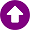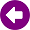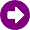﻿ Calculation method > ITU-R Rec. P.528

# ITU-R REC. P.528

Navegación:  Calculation method > ITU-R Rec. P.528

# ITU-R REC. P.528DESCRIPTION This model is based on Recommendation 528 of the ITU-R. It is a model that provides propagation curves for the aeronautical mobile and radionavigation using the VHF, UHF and SHF bands. It provides a method for predicting basic transmission loss in the frequency range 125 MHz to 15500 MHz for aeronautical and satellite services. The method is based on interpolation from empirically derived basic transmission loss curves as functions of distance, antenna height, frequency and percentage time. These sets of curves are valid for ground-air, ground-satellite, air-air, air-satellite, and satellite-satellite links. DEVELOPMENT The equivalent field strength for a given basic transmission loss is determined by:  E = 139,3 − Lt + 20 log(f) dB (μV/m) where: E: field strength (dB (μV/m)) for 1 kW e.r.p. Lt: transmission loss (dB) f: frequency (MHz) Transmission loss is obtained by means of an interpolation process as a function of distance, antenna height, frequency and percentage time. For these curves, it should be noted: The aeronautical curves are contained in Figs 1-1 to 8-5. These figures show median values of the basic transmission loss, Lb, for  time availabilities of 1, 5, 10, 50 and 95% at the frequencies 125, 300, 600, 1200, 2400, 5100, 9400 and 15500 MHz. For example, Lb(0.95) = 200 dB means that the basic transmission loss would be 200 dB or less during 95% of the time. Each figure consists of three curve sets representing basic transmission loss for receiver's height of 1000, 10000 and 20000 . The transmitter's antenna height vary from 1.5 m to 20000 m covering both ground station and aircraft heights. At zero distance, Lb(0.50) is simply the free-space value corresponding to a path length equal to the difference in antenna heights. The free-space curves shown were calculated for a 19985m height difference. An interpolation procedure is given in the ITU-R Rec. P.528-3 to calculate the basic transmission loss for non-standard values of h1 and h2 and distance d. If the frequency of interest is different from the normalized values, an interpolation procedure should be used for frequency data 125, 300, 600, 1200, 2400, 5100, 9400 and 15500 MHz to obtain basic transmission loss. If the time percentage of interest is different from the normalized values, an interpolation procedure is provided to calculate the basic transmission loss for any time percentage between 1% and 95%. All the procedures mentioned above have in common the use of different curves to obtain interpolation values. Once all of them have been applied, the resultant basic transmission loss value corresponds to the required distance, frequency, antennas height and time percentage. REFERENCES  ITU-R P.528-3, "Propagation curves for aeronautical mobile and radionavigation services using the VHF, UHF and SHF bands".